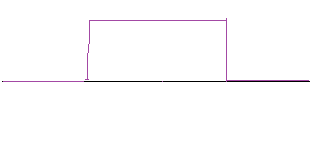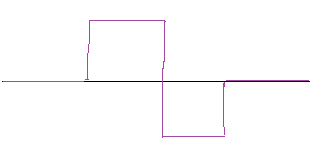## Monday, October 25, 2010

### How Cool are Wavelets

Ok, Todays topic, Wavelets.
Wavelets can be used to detect wave forms of some shape and frequency.
This is much like a poor man's FFT.

Wavlets work like this...
You have a 'template' wave shape that you are trying to detect.
For example a wave might look like this...This is a kind of random wave shape but lets assume it is important to detect when this wave form happens in some data stream.

The incoming data stream might look like this...The red wave to the right is the input data.
Hidden in there is the blue wave form with some noise.

What we do is convolute the input wave form with the wavelet. By convolute we mean take each point in the wavelet and multiply it by the corresponding point in the input data stream and do that for every possible position along the input data stream.
Thus we 'scan' the input stream with the wavelet generating a correlation factor for each point along the input data stream.

Like this...

`for i=0 to in.length sum[i] = 0; for k=0 to wavlet.length   sum[i] += in[i] * wavelet[k];`

Then the sum array will tell you how much a given point in the input correlates to the wavelet form.

If you study this algorithm you will see that where the wavelet is high and the input is high you get a large positive number. If the wavelet is negative and the input is negative you again get a large positive number.

If the input is zero and the wavelet is not zero
you still get zero, and also if the wavelet is zero and the input is not zero you still get zero.

If the input is the inverse of the wavelet then the
correlation will be negative.

A few notes: The wavelet must be normalized. The ideal is that the peak wavelet value is 1.0. Or you can make the integral of the wavelet be zero. It depends on what result you want.

The wavelet also has a frequency. You can stretch
or shrink the wavelet length to detect different frequencies of the wave form.

A specific frequency detector wavelet looks like this...This wavelet will detect a specific frequency. The more cycles in the wave the narrower the
frequency detection.

This wavelet is a low pass filter...And this is a high pass filter...The cool thing here is that the wavelet can detect a specific wave shape and timing without the more complex math of a complete FFT. Also with out knowing exact frequencies and such, you can detect a specific wave shape.

Very useful for heart beat detection, specific vibration modes, and many other uses.

There have been zillions of academic papers written analyzing specific wave shapes and exactly how they filter or detect frequencies. But to use wavelets it is not necessary to understand all the theory.

TF